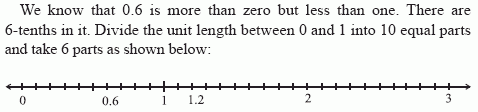Name: ___________________Date:___________________

 Email us to get an instant 20% discount on highly effective K-12 Math & English kwizNET Programs!

### MEAP Preparation - Grade 5 Mathematics1.72 Decimals on a Number Line

 Read the sequence of decimals given. Find the difference between the second and first decimal. Each succeeding decimal is the difference added to the previous decimal. Example 1: Complete the patters 0.6, 1.2, __Similarly 1.2 is represented. Second decimal - First decimal = 1.2 - 0.6 Difference = 0.6 Hence third decimal = second decimal + difference 1.2 + 0.6 = 1.8 Answer: 1.8 Example 2: Complete the patters 2.3, 2.5, ___, 2.7 Second decimal - First decimal = 2.5 - 2.3 Difference = 0.2 Hence third decimal = second decimal + difference 2.5 + 0.2 = 2.7 Answer: 2.7 Directions: Find the missing decimal in the pattern. Also write at least 10 examples of your own.Name: ___________________Date:___________________

### MEAP Preparation - Grade 5 Mathematics1.72 Decimals on a Number Line

 Q 1: Complete the pattern: 4.73, 4.731, 4.732, 4.733, ____, 4.735, 4.736, 4.737, 4.738, 4.739, 4.744.7354.7434.7344.733 Q 2: Complete the pattern: 18.76, 19.76, ____, 21.76, 22.7619.7721.7620.7619.86 Question 3: This question is available to subscribers only! Question 4: This question is available to subscribers only!